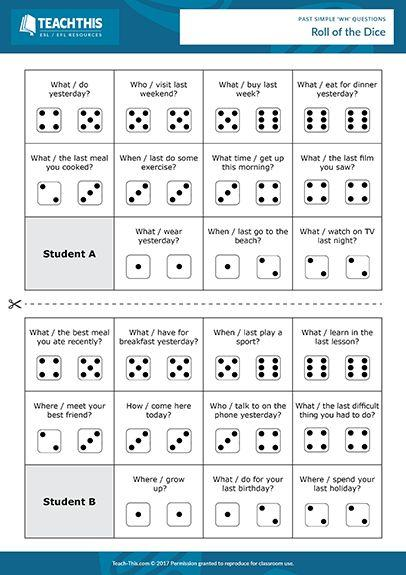STARTBODY

# Past Simple Wh Questions Roll of the Dice

Here's a fun past simple Wh questions ESL game to help you teach your students how to make questions in the past simple tense.To play the game, divide the students into pairs (A and B) and give each student a corresponding part of the worksheet and each pair two dice.

Student B begins by rolling the dice and adding up the numbers rolled.

Student A then formulates the past simple question that corresponds to the number on their worksheet.

For example, if Student B rolls a two, Student A asks question two on their worksheet, i.e. 'What did you wear yesterday?'

If Student B answers correctly, Student A crosses off the question. If Student B makes a grammatical mistake or is unable to answer appropriately in the form of a sentence, the question remains active and can be asked again, depending on the number rolled.

This process continues with students taking it in turns to roll the dice and ask and answer questions.

The first student to correctly answer all their partner's questions wins the game.

;
ENDBODY